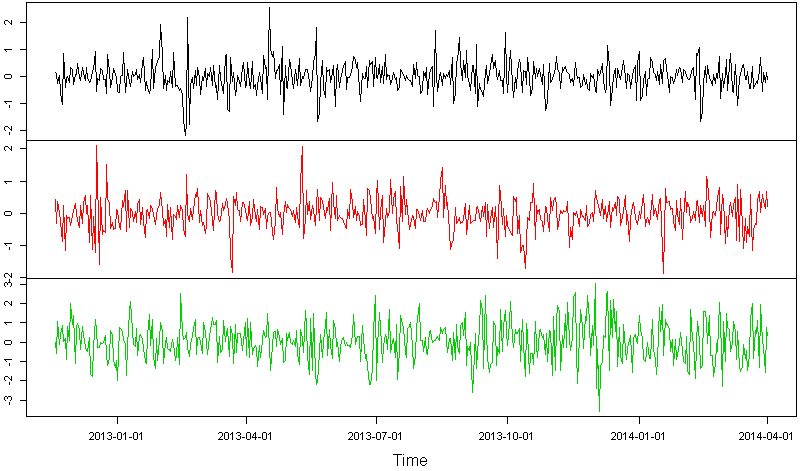# Development of new statistical methods for multivariate and dependent time series

M. Matilainen, K. Nordhausen, H. Oja

Blind Source Seperation (BSS) models are semiparametric models, where the components of a p-variate vector x (observed) are assumed to be a linear combinations of the components of some unobserved p-variate source vector z. In time series context, the observations are assumed to be from a p-variate time series.

In order to use these models, we need some assumptions for the unobserved vector z. For example, in independent component analysis (ICA) the components of z are assumed to be mutually independent, whereas in second order blind source separation models they are only assumed to be stationary and uncorrelated.

The objective of this research is the development of new statistical methods for multivariate and dependent time series. New methods are applied to multivariate heretoscedastic time series in signal processing and financing, for example. The aim in this project is to provide tools to recover hidden structures, latent variables, or latent subspaces in the multivariate and dependent time series data. The asymptotic properties and robustification of our new estimators are examined as well.

The new procedures could be seen as extensions of the classical analysis tools such as principal component analysis (PCA), independent component analysis (ICA) and second order blind source separation.